Next: Projection Profile Cutting Up: Formula Parsers Previous: Modified Grammars

## Box Languages

A box language divides the input plane into areas based on the symbols found. For example, the rule for a summation includes a term that subdivides the input area, as in Figure 2.5.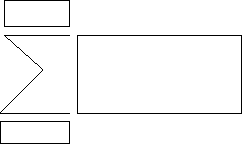When the parser finds a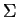'', it looks in the appropriate areas above and below the'' for the limits, then to the right for the expression being summed.

The way that box languages divide up the input area can be implemented in two ways. It can use explicit definitions in the grammar rules that specify where the boxes are, with respect to the symbol being considered. This approach is used by Anderson . An alternative approach is to use concatenation operators'' which offer geometric operations such as vertical concatenation''. Using concatenation operators, a fraction can recognised as a vertical concatenation of the numerator, a horizontal line and the denominator. This approach is used by Martin .

When the concatenation operators are applied, they define the subdivision of the input plane, restricting the positions in which to look for symbols. The way the concatenation operators actually subdivide the input area is defined externally, not as part of the grammar.

The use of box grammars is a common approach, from the early formula parsers through to systems currently being developed .

Martin's system from 1967  is one of the earliest systems for the parsing of mathematical formulae, processing handwritten formulae entered using a pen and tablet.

Martin gives details of the method he uses for analysing the formula on the 2D input plane, and how to decide where to look next for each part of the equation, based on these positional or concatenation'' operators. The rule for addition in Martin's grammar is (C= T* + E*). This defines the horizontal concatenation (C=) of a Term (T*) a plus symbol (+) and an Expression (E*).

Martin's system uses several specific hard-coded tests and rules in addition to the grammar, to ensure that his system works correctly. He constantly looks out two characters ahead for exponents on derivatives, e.g.: the i's in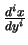. Each symbol input to the system has a bounding box that defines the area it covers. As the system works in a single left-to-right pass on the input, Martin needs to ensure that the correct symbols are encountered first. He extends the bounding boxes of's (for summation) and fraction bars (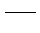) one character to the left. Doing so means that the symbol indicating the operation is found first, so when the symbols around it are found, the parser is able to associate them correctly with the operator.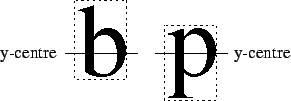Another well known early equation parser is Anderson's system . He assumes that the OCR problem has already been solved, so each character or syntactic unit'' has known physical bounds and an x- and y- centre, not necessarily the average of the left and right or upper and lower bounds. Working with these x- and y- centres avoids the problem of the bounding boxes of ascenders and descenders not lining up. Figuredemonstrates this. The bounding boxes are not lined up, however the y-centres of the symbols do.

To save on processing time, Anderson uses a preprocessing step to do an initial lexical analysis of the symbols input. Individual symbols are preassigned with their syntactic category, instead of using rules in the main grammar such as: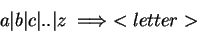Instead of using concatenation operators, Anderson puts tests in as part of rules in the grammar, limiting the positions where symbols can be legally positioned. As a result, the 2D rules in his grammar have several parts to them, that:

• check to see if the right syntactic units are present.

• check the layout of these syntactic units, using geometric constraints.

• have a rule to determine the physical characteristics of the result of applying the rule. This calculates things such as the size, position, and x- and y- centres of the new bounding box.

• build up a parse tree or a LISP-like expression that represents the formula.

The paper by Martin  discusses various aspects of input, parsing, and display of mathematical formulae. It provides useful results of a study they did on the syntax and layout of mathematical formulae. He determined that there is no one official'' layout, but he does come up with a number of general observations about the layout of formulae that hold true in most cases.

He discusses a method for parsing formulae, which is the same as that described by Martin in his earlier paper , and how to avoid problems with it. He also discusses the splitting of formulae across several lines when the formula is too long, display of formulae, ambiguity in input and how to judge if it is a valid expression.

More recent papers by Fateman et al.  and Fateman and Tokuyasu  discuss their recent work on the automatic interpretation of typeset formulae that have been scanned in from books of tables of integrals. Once interpreted, they intend to store the formulae so that they can be retrieved from an online integral lookup table, for use in computer algebra systems.

In order to simplify the problem, they assume that the layout of the typeset notations being input to the system is constant. This is a valid assumption, as all their input is being taken from a single book. They also assume that the output of the OCR system that feeds the recogniser is 100% correct, so the misrecognition errors are of no concern. Test input is currently cleaned up by hand if there are characters that have been mistakenly joined, or if there is excessive noise that is confusing the OCR stage.

Their formula parsing technique is similar to the box language used by Anderson . The input to their system is taken from the output of a sub-system that automatically processes raw bitmaps of scanned pages or screen-captures of previewed LATEX formulae. The sub-system automatically locates and recognises text in these images. This is then passed on to the formula parsing stages.

Initially, before the main parsing stage, they do a lexical analysis to collect up multi-character components. For example, cos'' would originally be passed as three separate characters, i.e.: c'', o'' and s''. These are put together to make a single cos'' element. Other multi-element symbols are gathered up as well, such as ='' and i'', should this not have happened already in the preceding OCR stage. The main parsing stage then takes this and turns it into a description of the symbols' layout using positional operators. This is then parsed to generate a representation of the mathematical formula.

. The input to their system is taken from the output of a sub-system that automatically processes raw bitmaps of scanned pages or screen-captures of previewed LATEX formulae. The sub-system automatically locates and recognises text in these images. This is then passed on to the formula parsing stages.

Initially, before the main parsing stage, they do a lexical analysis to collect up multi-character components. For example, cos'' would originally be passed as three separate characters, i.e.: c'', o'' and s''. These are put together to make a single cos'' element. Other multi-element symbols are gathered up as well, such as ='' and i'', should this not have happened already in the preceding OCR stage. The main parsing stage then takes this and turns it into a description of the symbols' layout using positional operators. This is then parsed to generate a representation of the mathematical formula.Next: Projection Profile Cutting Up: Formula Parsers Previous: Modified Grammars
Steve Smithies
1999-11-13